# CIE A Level Chemistry复习笔记1.5.4 Enthalpy & Bond Energies

### Enthalpy & Bond Energies

• During a reaction, enthalpy changes take place because bonds are being broken and formed
• Energy (in the form of heat) is needed to overcome attractive forces between atoms
• Bond breaking is therefore endothermic
• Energy is released from the reaction to the surroundings (in the form of heat) when new bonds are formed
• Bond forming is therefore exothermic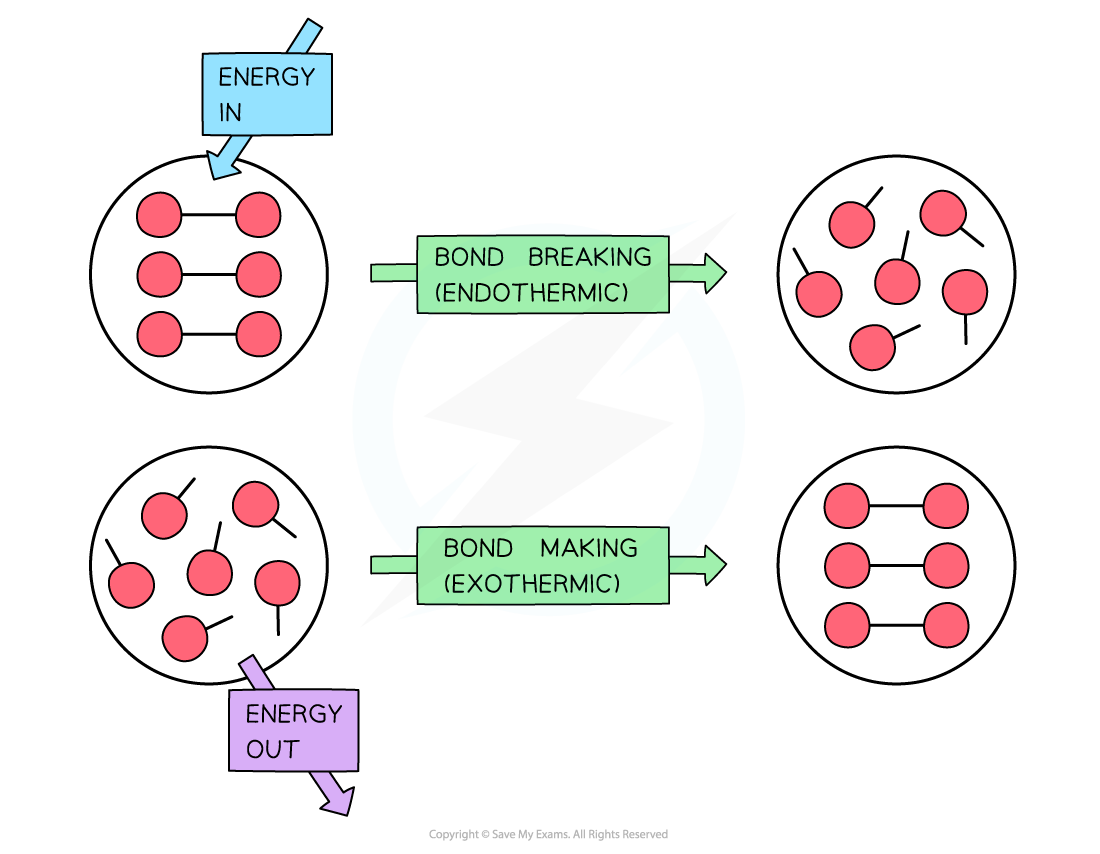To break bonds energy is required from the surroundings and to make new bonds energy is released from the reaction to the surroundings

• If more energy is required to break bonds than energy is released when new bonds are formed, the reaction is endothermic
• If more energy is released when new bonds are formed than energy is required to break bonds, the reaction is exothermic
• In reality, only some bonds in the reactants are broken and then new ones are formed

### Enthalpy Calculations

#### Exact bond energy

• The amount of energy required to break one mole of a specific covalent bond in the gas phase is called the bond dissociation energy
• Bond dissociation energy (E) is also known as exact bond energy or bond enthalpy
• The type of bond broken is put in brackets after E
• Eg. EE(H-H) is the bond energy of a mole of single bonds between two hydrogen atoms

#### Average bond energy

• Bond energies are affected by other atoms in the molecule (the environment)
• Therefore, an average of a number of the same type of bond but in different environments is calculated
• This bond energy is known as the average bond energy
• Since bond energies cannot be determined directly, enthalpy cycles are used to calculate the average bond energy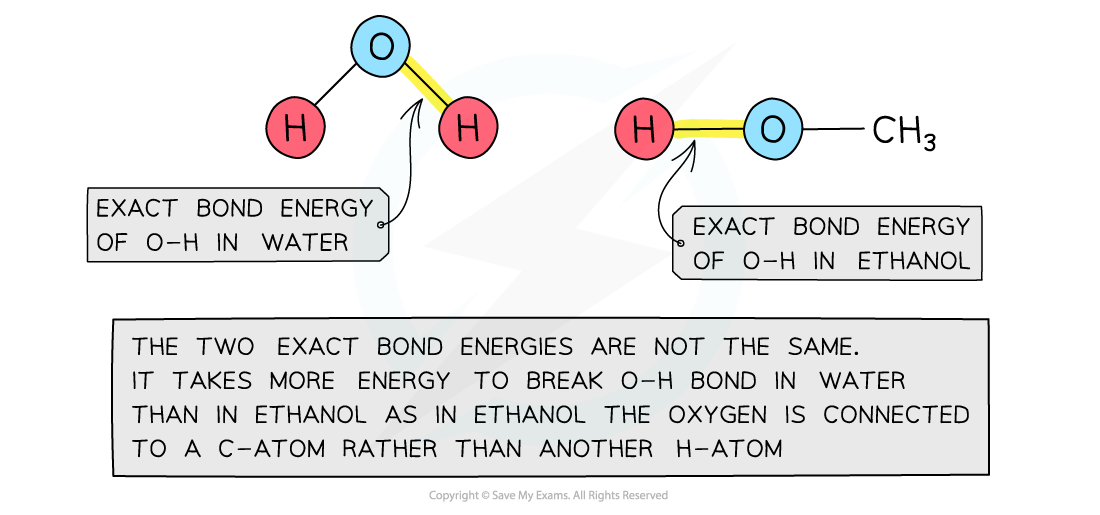Bond energies are affected by other atoms in the molecule

#### Calculating enthalpy change from bond energies

• Bond energies are used to find the ΔHrꝋ of a reaction when this cannot be done experimentally
• Eg. the Haber ProcessFormula of calculating the standard enthalpy change of reaction using bond energies

#### Worked example: Calculating the enthalpy change in the Haber process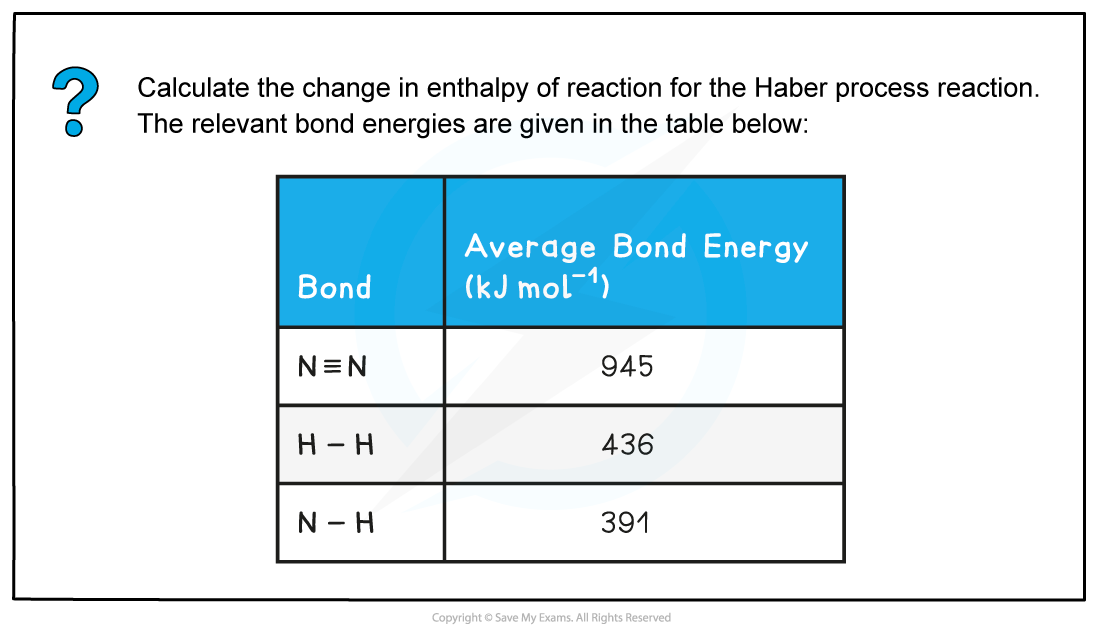• Step 1: The chemical equation for the Haber process is:

N2 (g) + 3H2 (g) ⇌ 2NH3 (g)

• Step 2: Set out the calculation as a balance sheet as shown below: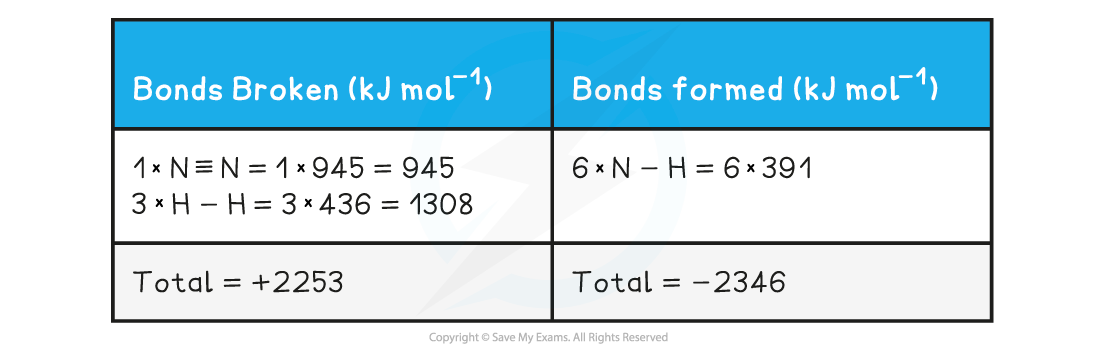Note! Values for bonds broken are positive (endothermic) and values for bonds formed are negative (exothermic)

• Step 3: Calculate the standard enthalpy of reaction

ΔHrꝋ = enthalpy change for bonds broken + enthalpy change for bonds formed= (+2253 kJ mol-1) + (-2346 kJ mol-1)= -93 kJ mol-1

#### Worked example: Calculating the average bond enthalpy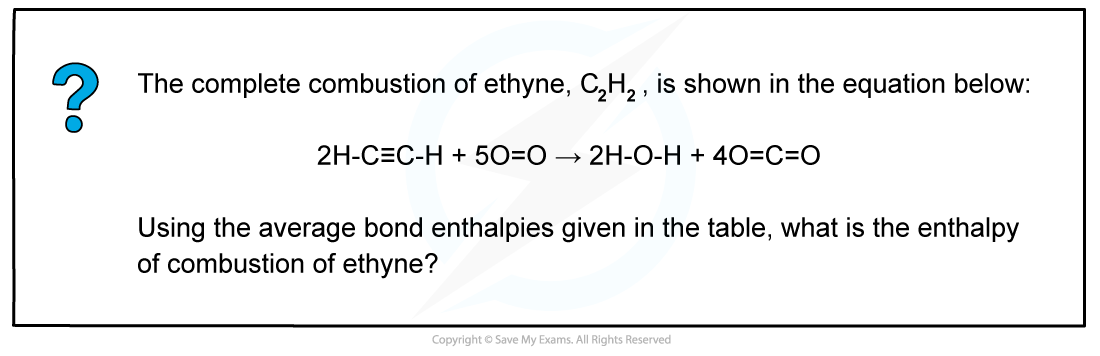• Step 1: The enthalpy of combustion is the enthalpy change when one mole of a substance reacts in excess oxygen to produce water and carbon dioxide

The chemical reaction should be therefore simplified such that only one mole of ethyne reacts in excess oxygen:

H-C=C-H + 2 ½ O=O → H-O-H + 2O=C=O

• Step 2: Set out the calculation as a balance sheet as shown below:ΔHrꝋ = enthalpy change for bonds broken + enthalpy change for bonds formed= (+2912 kJ mol-1) + (- 4142 kJ mol-1)= -1230 kJ mol-1

#### Measuring enthalpy changes

• Calorimetry is a technique used to measure changes in enthalpy of chemical reactions
• A calorimeter can be made up of a polystyrene drinking cup, a vacuum flask or metal canA polystyrene cup can act as a calorimeter to find enthalpy changes in a chemical reaction

• The energy needed to increase the temperature of 1 g of a substance by 1 oC is called the specific heat capacity (c) of the liquid
• The specific heat capacity of water is 4.18 J g-1 oC-1
• The energy transferred as heat can be calculated by:Equation for calculating energy transferred in a calorimeter

#### Worked example: Specific heat capacity calculations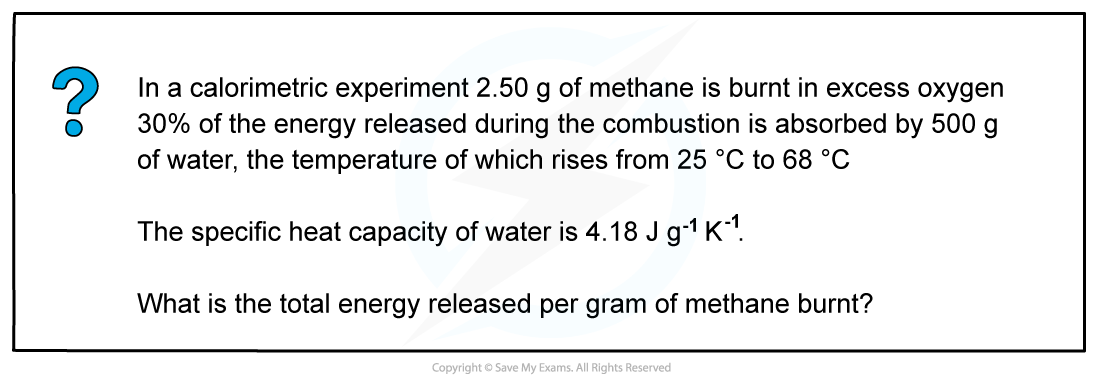• Step 1: q = m x c x ΔT

m (of water) = 500 g

c (of water) = 4.18 J g-1 K-1

ΔT (of water) = 68 oC - 25 oC

= 43 oC

= 43 K

The change in temperature in oC is equal to the change in temperature in K

• Step 2: q = 500 x 4.18 x 43

= 89 870 J

• Step 3:  This is only 30% of the total energy released by methane

Total energy x 0.3 = 89 870 J

Total energy = 299 567 J

• Step 4:  This is released by 2.50 g of methane= 119 827 J

= 120 kJ

#### Exam Tip

When new bonds are formed the amount of energy released is equal to the amount of energy absorbed when the same bonds are broken.For example:O2 (g) → 2O (g)           E (O=O) = +498 kJ mol-12O (g) → O2 (g)           E (O=O) + -498 kJ mol-1       Aqueous solutions of acid, alkalis and salts are assumed to be largely water so you can just use the m and c values of water when calculating the energy transferred. To then calculate any changes in enthalpy per mole of a reactant or product the following relationship can be used:When there is a rise in temperature, the value for ΔH becomes negative suggesting that the reaction is exothermic and when the temperature falls, the value for ΔH becomes positive suggesting that the reaction is endothermic.Also, remember that the ΔT is the same in oC and K!